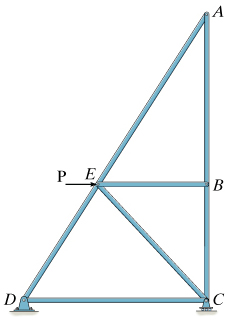### Create an Account

Home / Questions / To be able to identify zero-force members and understand their importance in truss analysi...

# To be able to identify zero-force members and understand their importance in truss analysis Zero-force members occur frequently in trusses and are typically used either to stabilize a truss during

To be able to identify zero-force members and understand their importance in truss analysis.

Zero-force members occur frequently in trusses and are typically used either to stabilize a truss during construction or to carry transient loads. Truss analysis can be greatly simplified by identifying and, subsequently, eliminating zero-force members.

Zero-force members

Zero-force members are truss members that carry no load under a given load configuration. Because they carry no load and their weight is negligible, compared with the loads carried by the truss, zero-force members experience no force. These members can be identified by drawing a free-body diagram of each joint and applying the conditions

?Fx=0 ?Fy=0

where x and y refer to the coordinate system applied at each joint.

Application of the equilibrium conditions leads to a simple method for identifying the zero-force members in planar trusses:

If the only members of an unloaded joint are two noncollinear members, both members are zero-force members.

If an unloaded joint has two collinear members and one noncollinear member only, the noncollinear member is a zero-force member.

Part A - Identifying zero-force members

Consider the truss shown below. Identify the zero-force members.Check all that apply.

 (1) AB (2) DE (3) CE (4) BE (5) AE (6) BC (7) CD

Show transcribed image text Zero-Force Members Learning Goal: To be able to identify zero-force members and understand their importance in truss analysis. Zero-force members occur frequently in trusses and are typically used either to stabilize a truss during construction or to carry transient loads. Truss analysis can be greatly simplified by identifying and, subsequently, eliminating zero-force members. Zero-force members Zero-force members are truss members that carry no load under a given load configuration. Because they carry no load and their weight is negligible, compared with the loads carried by the truss, zero-force members experience no force. These members can be identified by drawing a free-body diagram of each joint and applying the conditions ?Fx=0 ?Fy=0 where x and y refer to the coordinate system applied at each joint. Application of the equilibrium conditions leads to a simple method for identifying the zero-force members in planar trusses: If the only members of an unloaded joint are two noncollinear members, both members are zero-force members. If an unloaded joint has two collinear members and one noncollinear member only, the noncollinear member is a zero-force member. Part A - Identifying zero-force members Consider the truss shown below. Identify the zero-force members. Check all that apply.

May 13 2020 View more View LessSubscribe To Get Solution